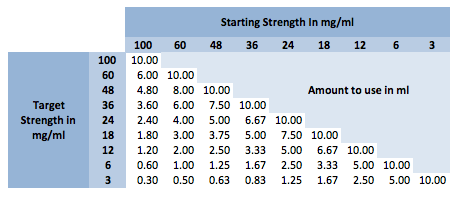Nicotine dilutions - with examples.

While there are calculators for e-Juice that include this, sometimes we just need to know how much NicBase to use because we want simple, easy and tasty juices fast.

This discussion is only going to address the physical dilution of Nicotine Liquids in milliliters (ml) as that is the most common way Do It Yourself (DIY) preparations are made. Most DIY users will not have or buy a scale and we can use weights as a topic later if need be.

The first thing to understand is the math. It's actually pretty simple but we will work it out here in an easy to follow and easy to understand format.

The formula is: “( TS/SS)*BS" or Target Strength(TS) / Starting Strength(SS) x Bottle Size(BS)

Let's use the following assumption to build a dilution:

• Target Strength (TS) 3mg/ml
• Starting Strength (SS) 100mg/ml
• Bottle Size (BS) 30ml

The resulting formula is: ( 3mg/100mg)*30ml

Working the formula looks like this:

( 3/100)*30 = (0.03)*30ml = 0.90ml (of 100mg/ml in a 30ml bottle)

This is true of any strength to lower strength you start with.

Another one (uncolored this time) is 18mg/ml to 6mg/ml in a 10ml bottle.

Formula: (6/18)*10 = (0.3333)*10 = 3.33ml in a 10ml bottle.

Here is a chart that does this for you in 10ml bottles. We used 10ml because you can easily multiply (or divide) for any other bottle size. Remember this ONLY takes into account the dilution not the base ratio. Also, if doing the math manually you have to remember to do the values in the parenthesis first, then multiply by the bottle size.Happy Vaping